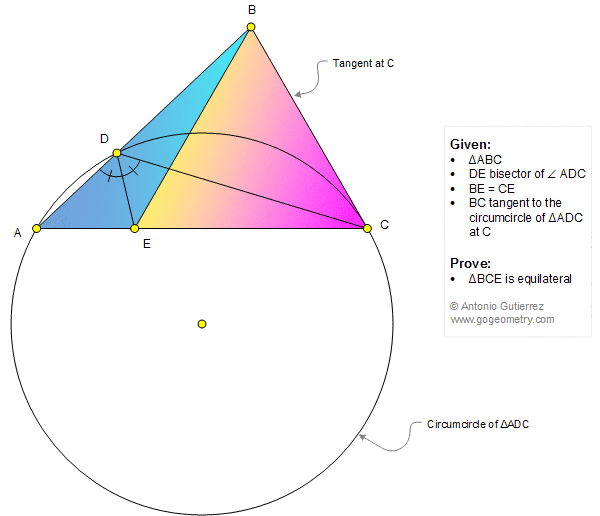# Math Infographic, Geometry Problem 1347: Triangle, Angle Bisector, Congruence, Circumcircle, Tangent, Equilateral Triangle

### Proposition

The figure shows a triangle ABC with DE bisector of angle ADC, BE = CE and BC tangent to the circumcircle of triangle ADC at C. Prove that the triangle BCE is equilateral.